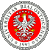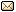XXXIX Workshop on Geometric Methods in Physics 27.06-3.07.2021 IX School on Geometry and Physics 21-25.06.2021
 Home First Announcement Travel and local information Lecturers (WGMP) Lecturers (SGP) Registration Photos Proceedings Previous Workshops Email

# Ashis Saha

## Holographic entanglement entropy and generalized entanglement temperature

In this work we study the flow of holographic entanglement entropy in dimensions $d \geq 3$ in the gauge/gravity duality set up. We observe that a generalized entanglement temperature $T_g$ can be defined which gives the Hawking temperature $T_H$ in the infrared region and leads to a generalized thermodynamics like law $E= \left(\frac{d-1}{d}\right)T_g~S_{REE}$, which becomes an exact relation in the entire region of the subsystem size $l$, including both the infrared ($l\rightarrow\infty$) as well as the ultraviolet ($l\rightarrow 0$) regions. Furthermore, in the IR limit, $T_g$ produces the Hawking temperature $T_H$ along with some correction terms which bears the signature of short distance correlations along the entangling surface. Moreover, for $d\geq 3$, the IR limit of the renormalized holographic entanglement entropy gives the thermal entropy of the black hole as the leading term, however, does not have a logarithmic correction to the leading term unlike the BTZ black hole ($d=2$) case. The generalized entanglement temperature $T_g$ also firmly captures the quantum mechanical to thermal crossover in the dual field theory at a critical value $l_c$ of the subsystem size in the boundary which we graphically represent for $AdS_{3+1}$ and $AdS_{4+1}$ black holes. We observe that this critical value $l_c$ where the crossover takes place decreases with increase in the dimension of the spacetime.

Comment: Based on the work: Phys. Rev. D 100, 106008 (2019).

 Event sponsored by: University of BialystokWebpage by: Tomasz Golinski